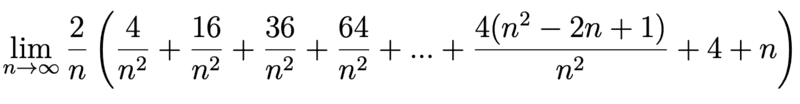# Identifying variables from Riemann sum limits

crememars
Homework Statement:
Consider the following limit of a Riemann sum for a function f on [a, b]. Identify f, a, and b,
and express the limit as a definite integral.

*see actual expression in the description below. it was too complicated to type out so I included a picture instead.
Relevant Equations:
∆x = (b-a)/n
xiR = a + i∆x
xiL = a + (i-1)∆xHi! I understand that this is an expanded Riemann sum but I'm having trouble determining its original form. I don't actually have any ideas as to how to find it, but I know that once I determine the original form of the Riemann sum, I will be able to figure out the values for a, b, and f.

If anyone could suggest how to proceed I would really appreciate it. Thank you :)

Gold Member
The last term is 2. For the other sums you shall use the formula
$$1^2+2^2+3^2+...+n^2=\frac{n(n+1)(2n+1)}{6}$$

•crememars
Homework Helper
2022 Award
Hi! I understand that this is an expanded Riemann sum but I'm having trouble determining its original form. I don't actually have any ideas as to how to find it, but I know that once I determine the original form of the Riemann sum, I will be able to figure out the values for a, b, and f.

If anyone could suggest how to proceed I would really appreciate it. Thank you :)

Compare the sum to $$\Delta x \sum_{i=1}^{n} f(a + i\Delta x).$$ What would be a good guess for $\Delta x$? One of the terms is given explicitly as $$\frac{4(n^2 - 2n + 1)}{n^2} =\frac{4(n-1)^2}{n^2}.$$ Can you guess the general form of the terms, and is your guess consistent with the first few terms given? How would you relate that general form to $f(a + i\Delta x)$?

•crememars
crememars
forgot to answer ! thank you for your help @pasmith and @anuttarasammyak :) I separated the terms and got f(x)= x^2 + 1

xiR = 2i/n -> x^2
n is just the riemann sum of 1 -> +1
∆x = 2/n

xiR = a + i∆x = a + 2i/n = 2i/n so a = 0 and b = 2